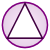Games
Problems
Go Pro!

# Rectangles and Squares

Pro Problems > Math > Geometry > Rectangles and Squares

# Featured Pro ProblemsAdjust the dimensions of a rectangle until the resulting figure is a squareCalculator may be helpful on this algebra intensive geometry problemA geometry problem involving areas and perimeters of squaresBuilding a room with specific dimension and area requirements - calculate the dimensionsFind the diagonal of a rectangle, given its area and perimeterHelp the farmer figure out how much fencing he needs for his fieldsFind the common side of a rectangle and a square.Calculate the perimeter of a rectangle, given information about changing dimensions

# Full Directory Listing

Area, Perimeter, Diagonal, Blue Square, Yellow Rectangle, Building a Room, Decreasing Width, Field Area, On the Edge, Overlapped Rectangles, Perimeter and Lengths, Rectangle and Square, Rectangle Diagonal, Splitting the Field, Stretching Rectangle, The Farmer in the DellLike us on Facebook to get updates about new resources